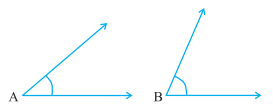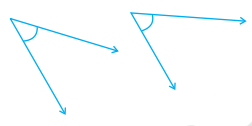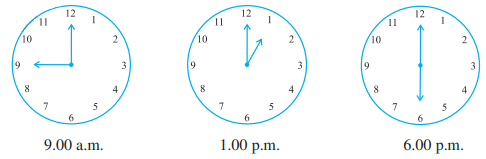### Chapter - 5 Understanding Elementary Shapes

Q
##### Understanding Elementary Shapes

Question:

1. What is the measure of (i) a right angle? (ii) a straight angle?

Solution: (i) The measure of a right angle is 900.

(ii) The measure of a straight angle is 1800.

2. Say True or False :

(a) The measure of an acute angle < 90°.

(b) The measure of an obtuse angle < 90°.

(c) The measure of a reflex angle > 180°.

(d) The measure of one complete revolution = 360°.

(e) If m A∠ = 53° and m B∠ = 35°, then m ∠A  > m ∠B.

Solution: (a) True.

(b) False.

(c) True.

(d) True.

(e) True.

3. Write down the measures of

(a) some acute angles.    (b) some obtuse angles.

(give at least two examples of each).

Solution: (a) The measure of an acute angle is less than 90°, for example: 30°, 45°.

(d) The measure of an obtuse angle is greater than 90°, for example: 140°, 105°.

4. Measure the angles given below using the Protractor and write down the measure.Solution: (a) The measure of an angle is 450.

(b) The measure of an angle is 1200.

(c) The measure of an angle is 900.

(d) The measure of an angle is 600, 1300 and 900.

5. Which angle has a large measure? First estimate and then measure.

Measure of Angle A =       Measure of Angle B =Solution: Measure of A = 45

Measure of B = 650

B has a large measure than A.

6. From these two angles which has larger measure? Estimate and then confirm by measuring them.Solution: By estimation the measure of second angle is larger.

Measure of angles are 45and 550.

7. Fill in the blanks with acute, obtuse, right or straight :

(a) An angle whose measure is less than that of a right angle is______.

(b) An angle whose measure is greater than that of a right angle is ______.

(c) An angle whose measure is the sum of the measures of two right angles is _____.

(d) When the sum of the measures of two angles is that of a right angle, then each one of them is ______.

(e) When the sum of the measures of two angles is that of a straight angle and if one of them is acute then the other should be _______.

Solution:

(a) An angle whose measure is less than that of a right angle is acute angle.

(b) An angle whose measure is greater than that of a right angle is obtuse angle.

(c) An angle whose measure is the sum of the measures of two right angles is straight angle.

(d) When the sum of the measures of two angles is that of a right angle, then each one of them is acute angle.

(e) When the sum of the measures of two angles is that of a straight angle and if one of them is acute then the other should be obtuse angle.

8. Find the measure of the angle shown in each figure. (First estimate with your eyes and then find the actual measure with a protractor).Solution: The measure of the angle shown in the figure are 400,1300,65and 1350 respectively.

9. Find the angle measure between the hands of the clock in each figure :Solution: The angle measure in the given clock are 900, 300 and 180respectively.

10. Investigate

In the given figure, the angle measures 30°. Look at the same figure through a magnifying glass. Does the angle becomes larger? Does the size of the angle change?Solution: No, the measure of angle remains the same through a magnifying glass.

11. Measure and classify each angle :Solution: